Ex 12.3

Chapter 12 Class 10 Areas related to Circles
Serial order wise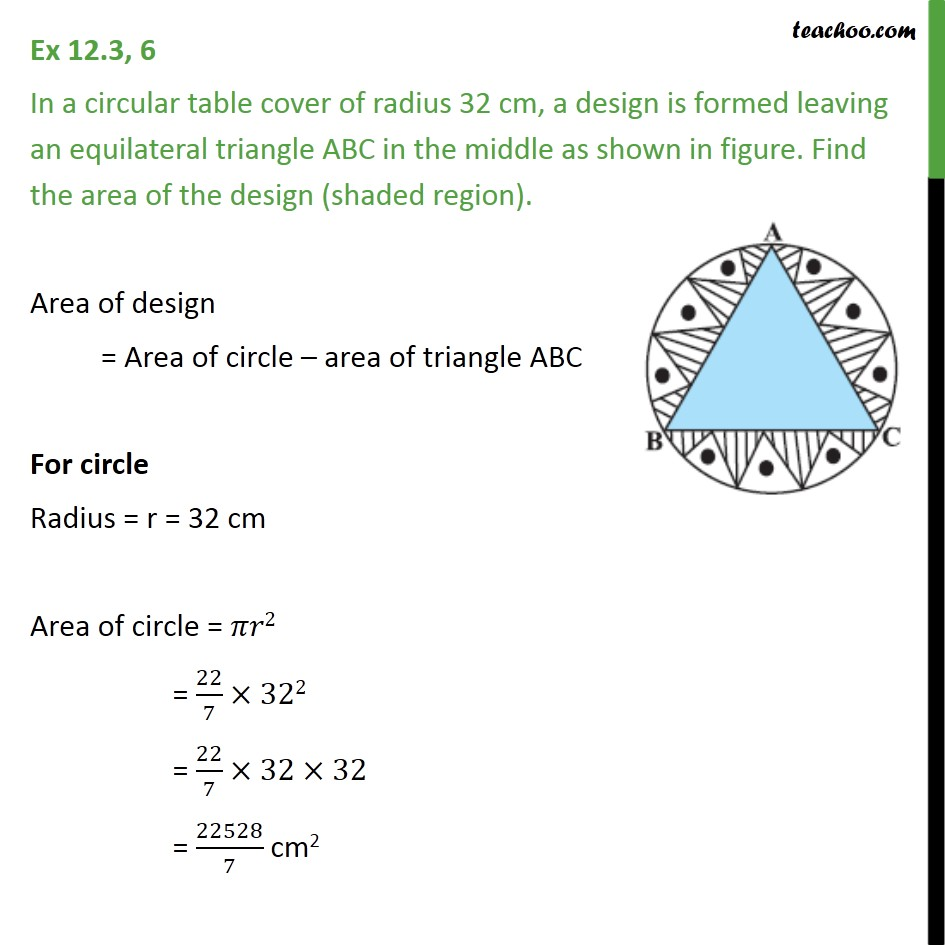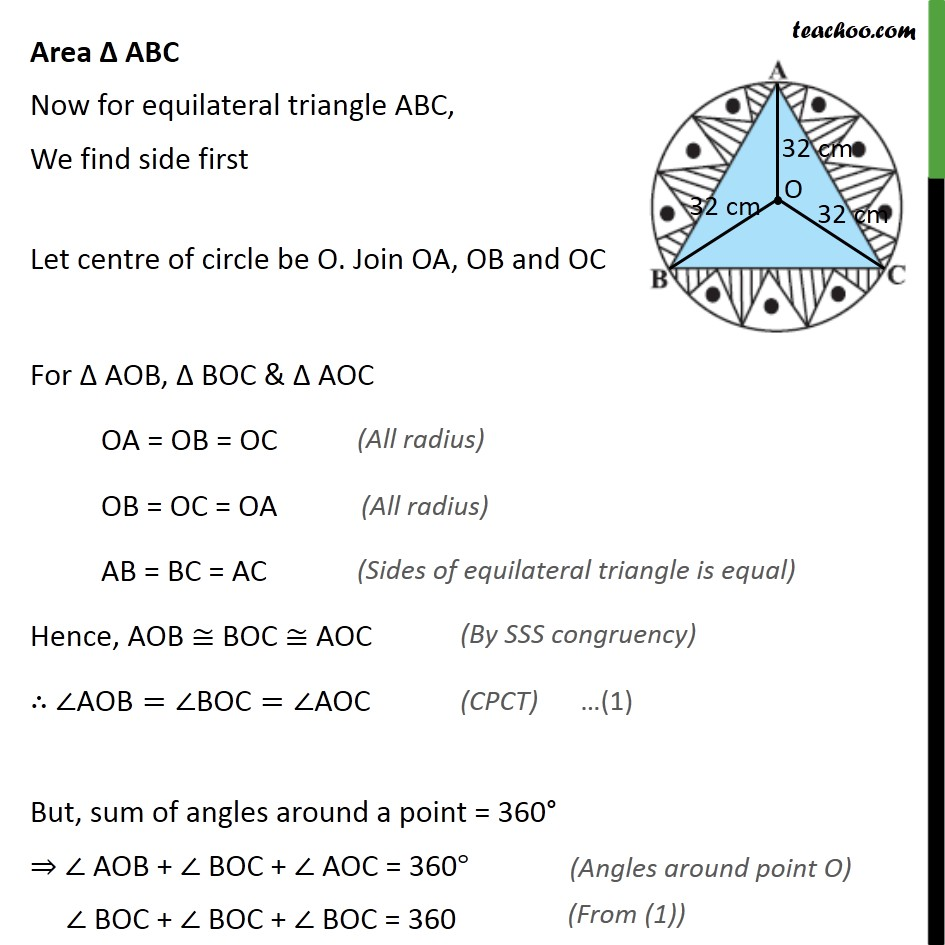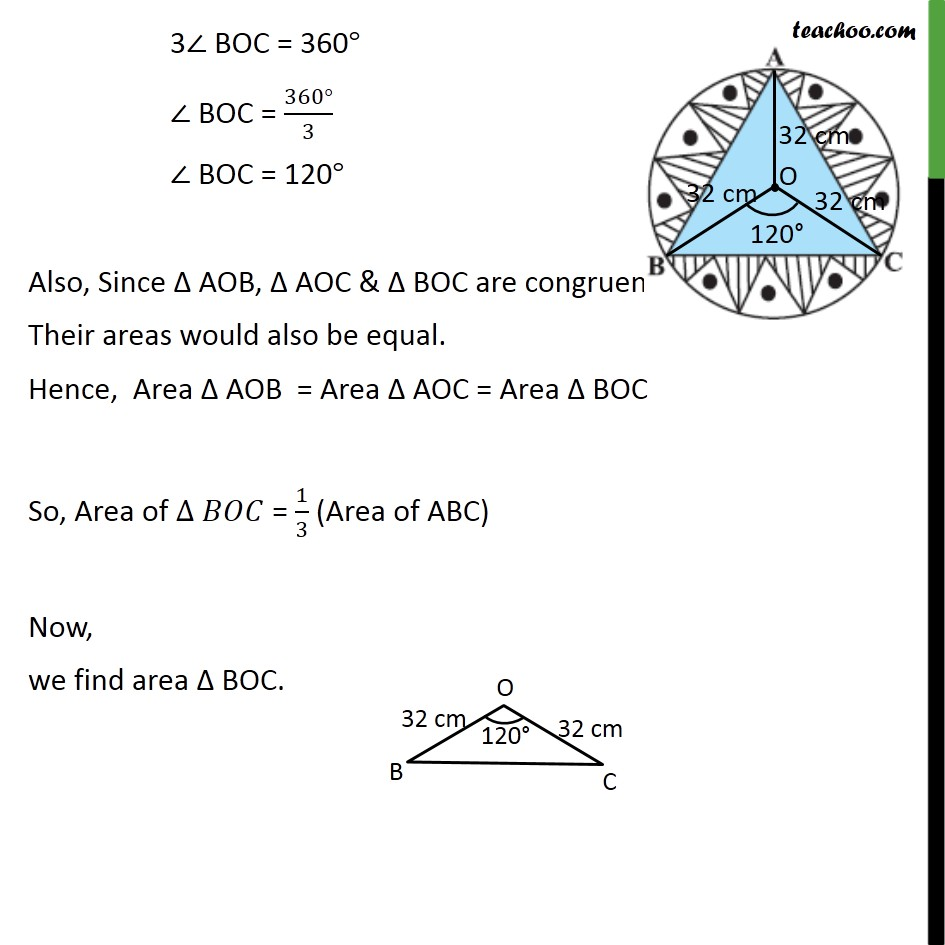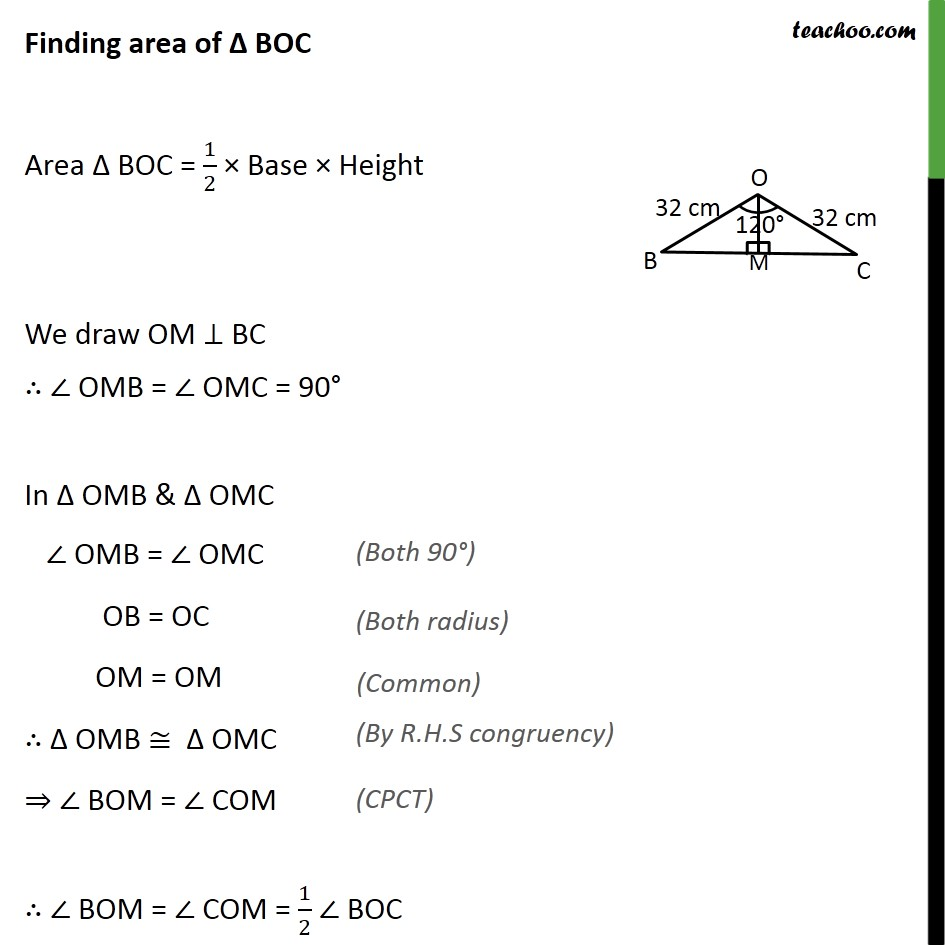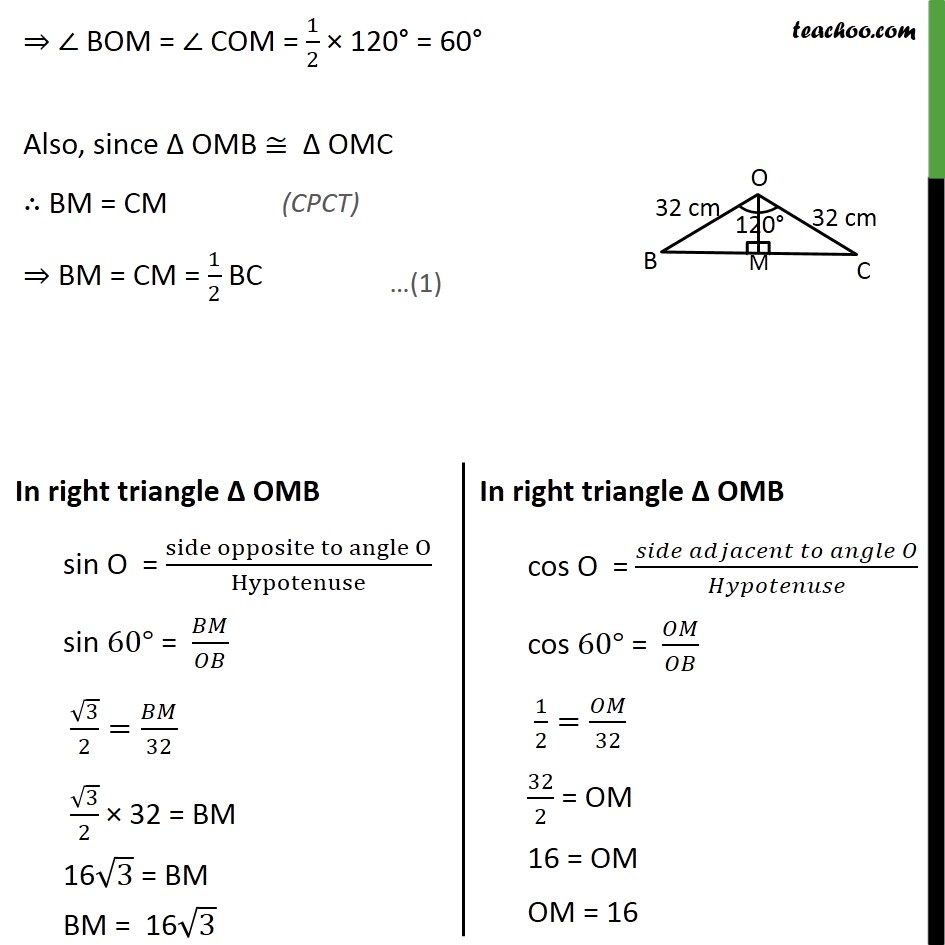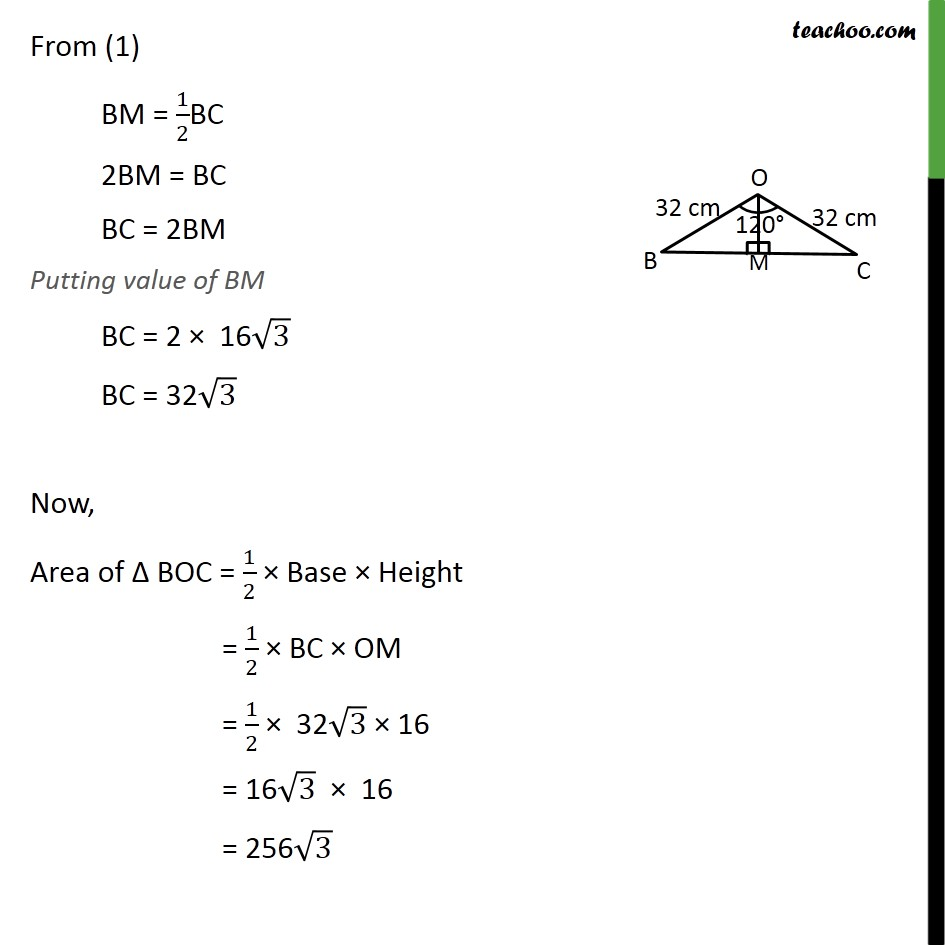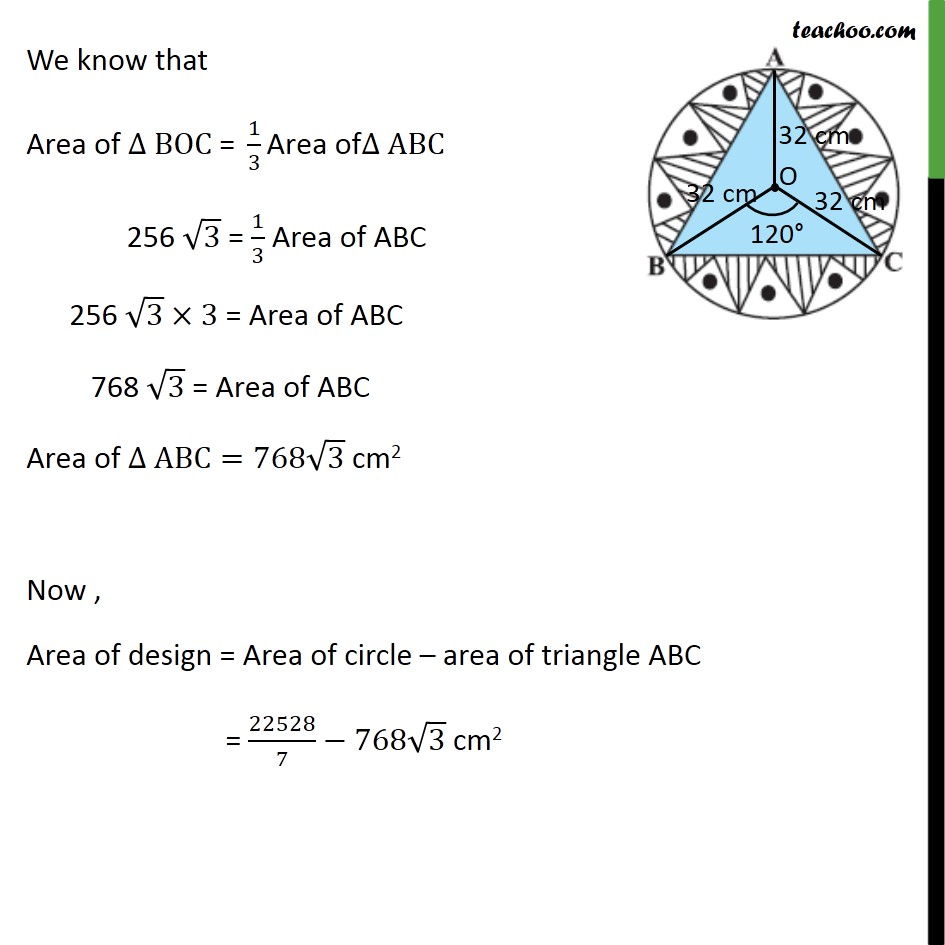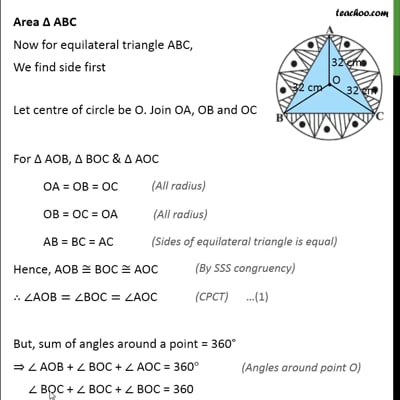This video is only available for Teachoo black users

Introducing your new favourite teacher - Teachoo Black, at only ₹83 per month

### Transcript

Ex 12.3, 6 In a circular table cover of radius 32 cm, a design is formed leaving an equilateral triangle ABC in the middle as shown in figure. Find the area of the design (shaded region). Area of design = Area of circle – area of triangle ABC For circle Radius = r = 32 cm Area of circle = 𝜋𝑟2 = 22/7×322 = 22/7×32×32 = 22528/7 cm2 Area Δ ABC Now for equilateral triangle ABC, We find side first Let centre of circle be O. Join OA, OB and OC For Δ AOB, Δ BOC & Δ AOC OA = OB = OC OB = OC = OA AB = BC = AC Hence, AOB ≅ BOC ≅ AOC ∴ ∠"AOB"=∠"BOC"=∠AOC But, sum of angles around a point = 360° ⇒ ∠ AOB + ∠ BOC + ∠ AOC = 360° ∠ BOC + ∠ BOC + ∠ BOC = 360 3∠ BOC = 360° ∠ BOC = (360°)/3 ∠ BOC = 120° Also, Since Δ AOB, Δ AOC & Δ BOC are congruent Their areas would also be equal. Hence, Area Δ AOB = Area Δ AOC = Area Δ BOC So, Area of ∆ 𝐵𝑂𝐶 = 1/3 (Area of ABC) Now, we find area Δ BOC. Finding area of Δ BOC Area Δ BOC = 1/2 × Base × Height We draw OM ⊥ BC ∴ ∠ OMB = ∠ OMC = 90° In Δ OMB & Δ OMC ∠ OMB = ∠ OMC OB = OC OM = OM ∴ Δ OMB ≅ Δ OMC ⇒ ∠ BOM = ∠ COM ∴ ∠ BOM = ∠ COM = 1/2 ∠ BOC ⇒ ∠ BOM = ∠ COM = 1/2 × 120° = 60° Also, since Δ OMB ≅ Δ OMC ∴ BM = CM ⇒ BM = CM = 1/2 BC From (1) BM = 1/2BC 2BM = BC BC = 2BM Putting value of BM BC = 2 × 16√3 BC = 32√3 Now, Area of Δ BOC = 1/2 × Base × Height = 1/2 × BC × OM = 1/2 × 32√3 × 16 = 16√3 × 16 = 256√3 We know that Area of ∆ BOC" = " 1/3 "Area of" ∆ ABC 256 √3 = 1/3 Area of ABC 256 √3×3 = Area of ABC 768 √3 = Area of ABC Area of ∆ ABC=768√3 cm2 Now , Area of design = Area of circle – area of triangle ABC = 22528/7−768√3 cm2